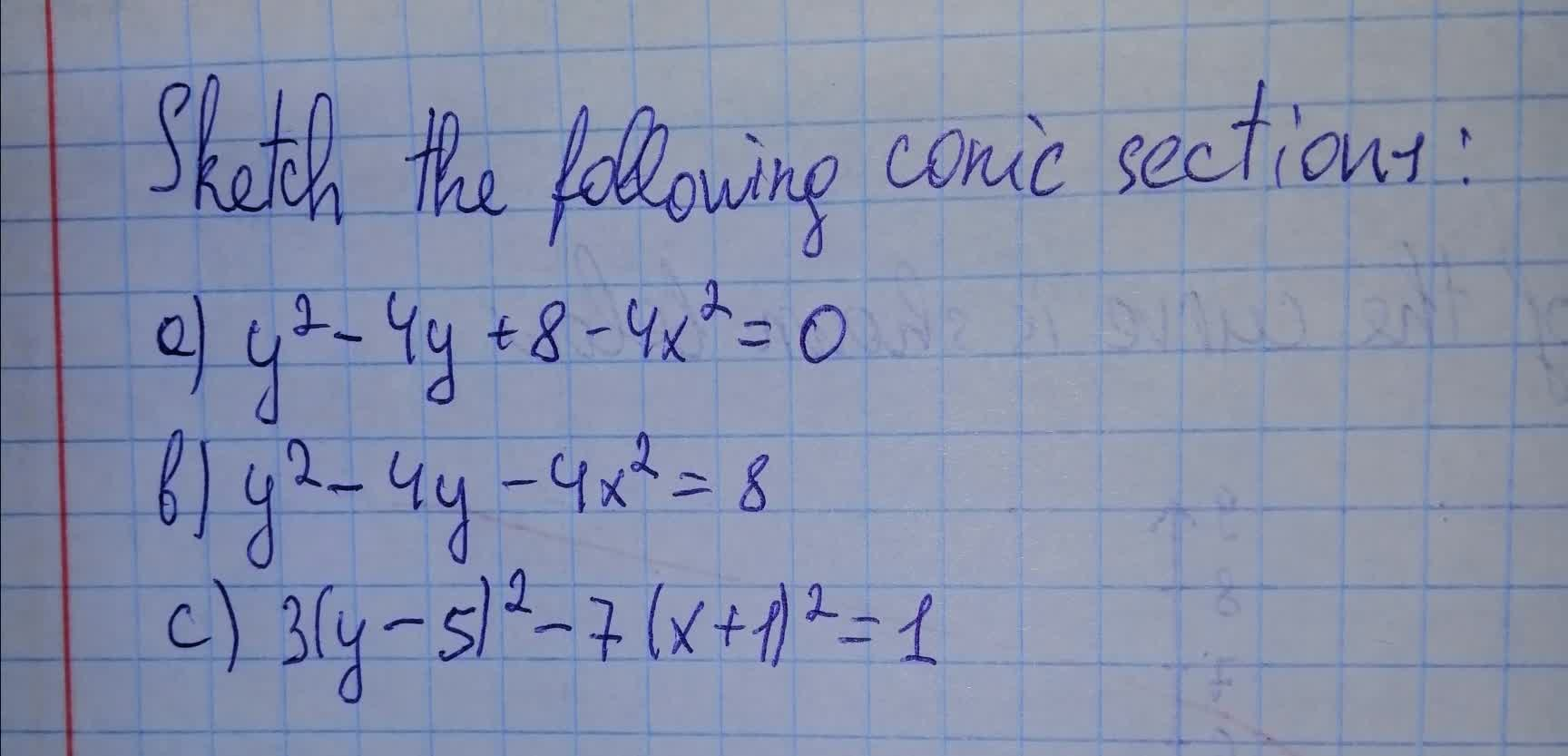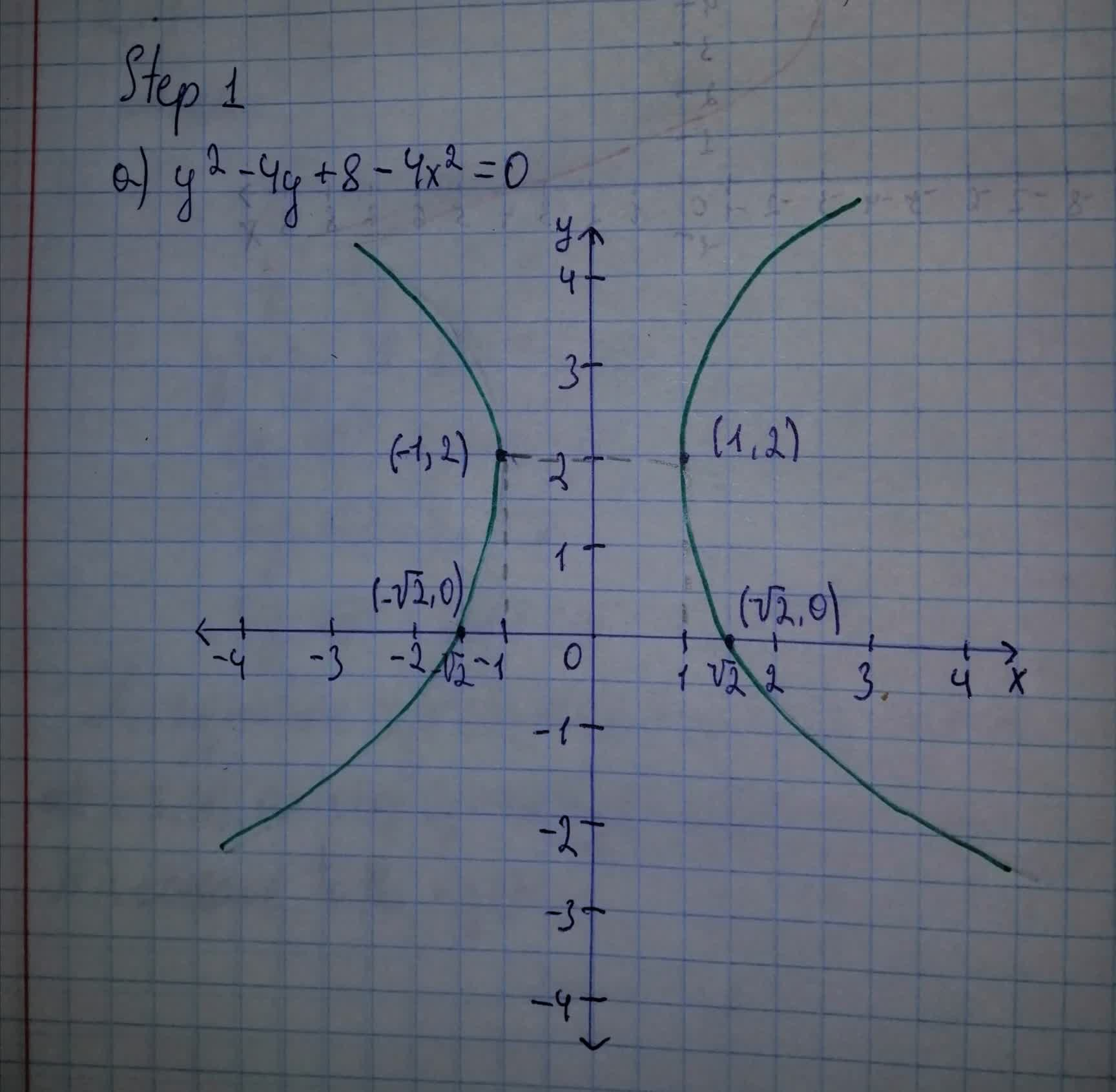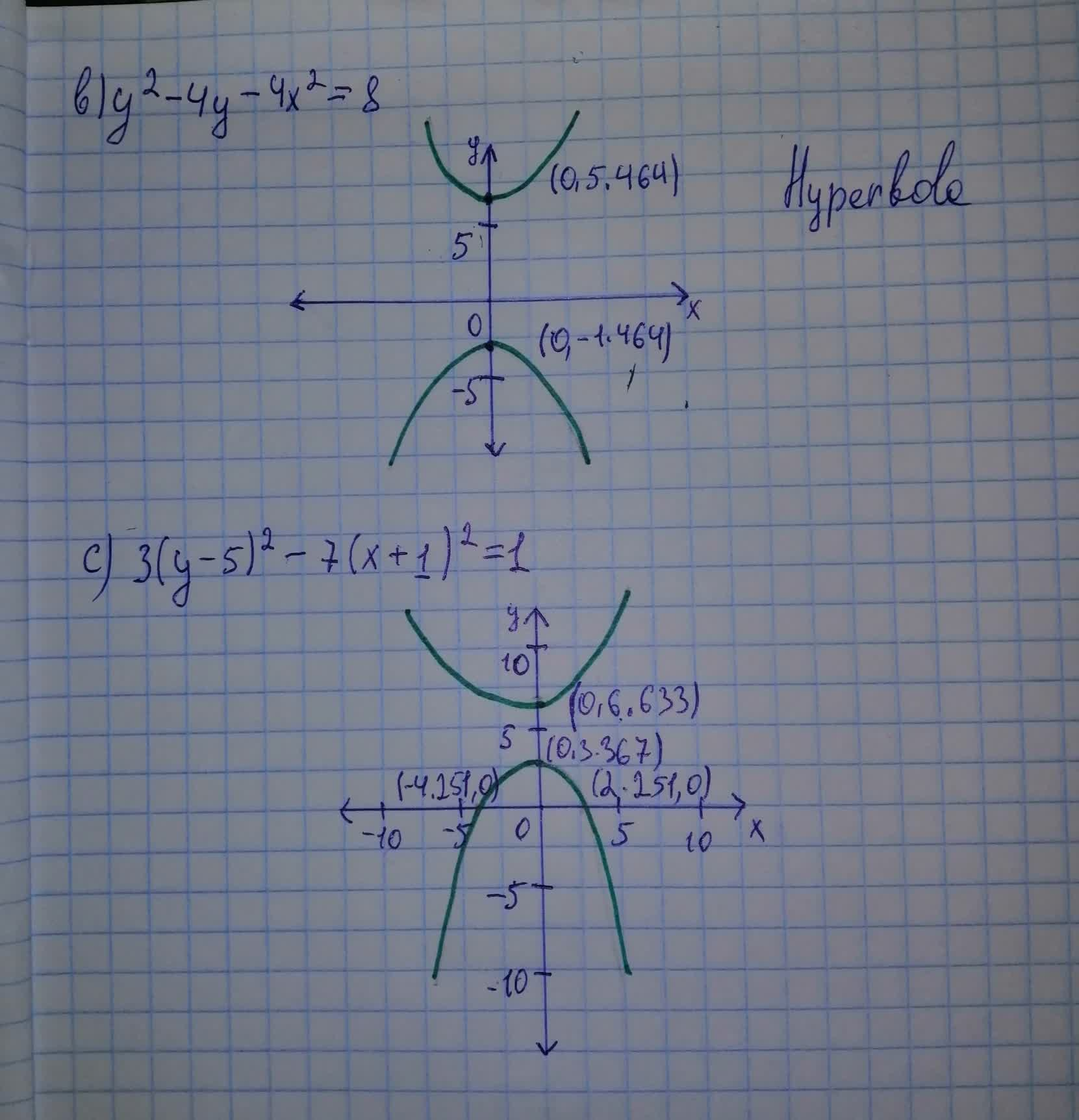# Sketch the following conic sections:a) y^2-4y+8-4x^2=8 b)y^2-4y-4x^2=8 c)3(y-5)^2-7(x+1)^2=1Maiclubk 2021-08-07 Answered
Sketch the following conic sections:
a)${y}^{2}-4y+8-4{x}^{2}=8$
b)${y}^{2}-4y-4{x}^{2}=8$
c)$3{\left(y-5\right)}^{2}-7{\left(x+1\right)}^{2}=1$You can still ask an expert for help

• Questions are typically answered in as fast as 30 minutes

Solve your problem for the price of one coffee

• Math expert for every subject
• Pay only if we can solve itNichole Watt

The answer is given below in the photo###### Not exactly what you’re looking for?

a)

Solve for y:
${y}^{2}-4y-4{x}^{2}+8=0$

Hint: Solve the quadratic equation by completing the square.
Subtract $-4{x}^{2}+8$ from both sides:
${y}^{2}-4y=4{x}^{2}-8$

Hint: Take one half of the coefficient of y and square it, then add it to both sides.
${y}^{2}-4y+4=4{x}^{2}-4$

Hint: Factor the left hand side.
Write the left hand side as a square:
$\left(y-2{\right)}^{2}=4{x}^{2}-4$

Hint: Eliminate the exponent on the left hand side.
Take the square root of both sides:
$y-2=\sqrt{4{x}^{2}-4}\phantom{\rule{1em}{0ex}}\text{or}\phantom{\rule{1em}{0ex}}y-2=-\sqrt{4{x}^{2}-4}$

Hint: Look at the first equation: Solve for y.
$y=\sqrt{4{x}^{2}-4}+2\phantom{\rule{1em}{0ex}}\text{or}\phantom{\rule{1em}{0ex}}y-2=-\sqrt{4{x}^{2}-4}$

Hint: Look at the second equation: Solve for y.
Answer: $y=\sqrt{4{x}^{2}-4}+2\phantom{\rule{1em}{0ex}}\text{or}\phantom{\rule{1em}{0ex}}y=2-\sqrt{4{x}^{2}-4}$

b)

Solve for y:
${y}^{2}-4y-4{x}^{2}=8$

Hint: Solve the quadratic equation by completing the square.
Add $4{x}^{2}$ to both sides:
${y}^{2}-4y=4{x}^{2}+8$

Hint: Take one half of the coefficient of y and square it, then add it to both sides.
${y}^{2}-4y+4=4{x}^{2}+12$

Hint: Factor the left hand side.
Write the left hand side as a square:
$\left(y-2{\right)}^{2}=4{x}^{2}+12$

Hint: Eliminate the exponent on the left hand side.
Take the square root of both sides:
$y-2=\sqrt{4{x}^{2}+12}\phantom{\rule{1em}{0ex}}\text{or}\phantom{\rule{1em}{0ex}}y-2=-\sqrt{4{x}^{2}+12}$

Hint: Look at the first equation: Solve for y.
$y=\sqrt{4{x}^{2}+12}+2\phantom{\rule{1em}{0ex}}\text{or}\phantom{\rule{1em}{0ex}}y-2=-\sqrt{4{x}^{2}+12}$

Hint: Look at the second equation: Solve for y.
Answer: $y=\sqrt{4{x}^{2}+12}+2\phantom{\rule{1em}{0ex}}\text{or}\phantom{\rule{1em}{0ex}}y=2-\sqrt{4{x}^{2}+12}$

c)

Solve for y:
$3{\left(y-5\right)}^{2}-7{\left(x+1\right)}^{2}=1$

Hint: Isolate terms with y to the left hand side.
Add $7\left(x+1{\right)}^{2}$ to both sides:
$3{\left(y-5\right)}^{2}=7{\left(x+1\right)}^{2}+1$

Hint: Divide both sides by a constant to simplify the equation.
Divide both sides by 3:
${\left(y-5\right)}^{2}=\frac{7}{3}{\left(x+1\right)}^{2}+\frac{1}{3}$

Hint: Eliminate the exponent on the left hand side.
Take the square root of both sides:
$y-5=\sqrt{\frac{7}{3}{\left(x+1\right)}^{2}+\frac{1}{3}}\phantom{\rule{1em}{0ex}}\text{or}\phantom{\rule{1em}{0ex}}y-5=-\sqrt{\frac{7}{3}{\left(x+1\right)}^{2}+\frac{1}{3}}$

Hint: | Look at the first equation: Solve for y.